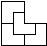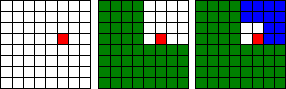# Golomb's inductive proof of a tromino theorem

Polyominos were invented by Solomon Golomb, then a 22 year-old student at Harvard, in 1954. A polyomino is a "rook"-connected set of equal squares. (In computer science the kind of connectedness where neighboring squares are required to share an edge is also known as the 4-connectedness. If two squares that share a vertex are also considered neighbors, we get the 8-connectedness.)

Trominos are polyominos that consist of three squares. There are two kinds of trominos: a 3×1 rectangle and an L-shaped piece. A property of the latter is the subject of the following theorem:

### Theorem

A unit square has been removed from a 2n×2n board. Prove that the rest of the board can be tiled with L-shaped trominos.

The applet below may help you grasp a proof that is quintessential mathematical induction. You may click inside the applet to "remove" a square or on the "One step" button, to see how, in applet's view, the proof may evolve. Exponent n could be changed with a spin control at the bottom of the applet.

### This applet requires Sun's Java VM 2 which your browser may perceive as a popup. Which it is not. If you want to see the applet work, visit Sun's website at https://www.java.com/en/download/index.jsp, download and install Java VM and enjoy the applet.

 What if applet does not run?

Proof### Proof

The proof is by induction. For n = 1, the assertion is obvious. With a square removed from a 2×2 board, the remaining part is exactly an L-shaped tromino piece.

Assume the statement true for n = k-1 and let n = k. Draw the center lines of the 2k×2k board. The cut-off square lies in exactly one of the four thus obtained 2k-1×2k-1 boards. We may remove one square from each of the other three boards by placing a tromino at the center of the 2k×2k board. The result is a tromino and four 2k-1×2k-1 boards, each with a square removed - just a situation to apply the inductive hypothesis.

The proof is so simple, its first step was made into a proof without words.

### Remark 1

You can test your understanding of Golomb's theorem by trying to tile the board manually.

### Remark 2

We may base the last step of the proof on the fact that a tromino can be covered with 4 twice as small copies of itself which makes it a rep-4 tile (a rep-tile variety):Thus a 2k×2k board could be looked at as combining one big piece (green) of tromino and a smaller, 2k-1×2k-1 board with a 1×1 square removed.### References

1. B. Bollobás, The Art of Mathematics: Coffee Time in Memphis, Cambridge University Press, 2006, #40
2. S. Golomb, Two Right Tromino Theorems, in The Changing Shape of Geometry, edited by C. Pritchard, Cambridge University Press, 2003
3. R. Nelsen, Proofs Without Words II, MAA, 2000, p. 123• Covering A Chessboard With Domino
• Dominoes on a Chessboard
• Tiling a Chessboard with Dominoes
• Vertical and Horizontal Dominoes on a Chessboard
• Straight Tromino on a Chessboard
• Tromino Puzzle: Interactive Illustration of Golomb's Theorem
• Tromino as a Rep-tile
• Tiling Rectangles with L-Trominoes
• Squares and Straight Tetrominoes
• Covering a Chessboard with a Hole with L-Trominoes
• Tromino Puzzle: Deficient Squares
• Tiling a Square with Tetrominoes Fault-Free
• Tiling a Square with T-, L-, and a Square Tetrominoes
• Tiling a Rectangle with L-tetrominoes
• Tiling a 12x12 Square with Straight Trominoes
• Bicubal Domino
•